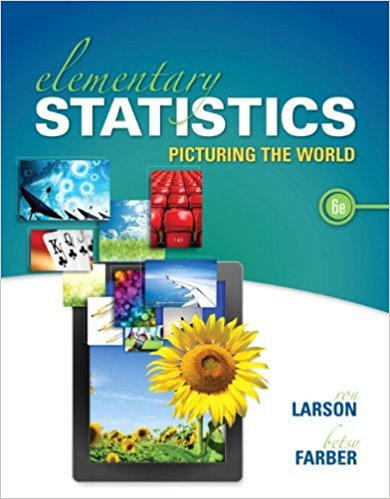×
Get Full Access to Elementary Statistics: Picturing The World - 6 Edition - Chapter 4.3 - Problem 23e
Get Full Access to Elementary Statistics: Picturing The World - 6 Edition - Chapter 4.3 - Problem 23e

×

# Using a Distribution to Find Probabilities In | Ch 4.3 - 23EISBN: 9780321911216 66

## Solution for problem 23E Chapter 4.3

Elementary Statistics: Picturing the World | 6th Edition

• Textbook Solutions
• 2901 Step-by-step solutions solved by professors and subject experts
• Get 24/7 help from StudySoup virtual teaching assistantsElementary Statistics: Picturing the World | 6th Edition

4 5 1 301 Reviews
23
5
Problem 23E

Problem 23E

Using a Distribution to Find Probabilities In Exercise, find the indicated probabilities using the geometric distribution, the Poisson distribution, or the binomial distribution. Then determine whether the events are unusual. If convenient, use a table or technology to find the probabilities.

Education Fifty-four percent of parents would give up cable television to have their child’s education paid for. You randomly select five parents. Find the probability that the number of parents who would give up cable television to have their child’s education paid for is (a) exactly three, (b) less than four, and (c) at least three.

Step-by-Step Solution:

Step 1 of 1

(a)

We are asked to find the indicated probabilities using the geometric distribution, the Poisson distribution, or the binomial distribution.

Then determine whether the events are unusual.

If convenient, use a table or technology to find the probabilities.

We have given 54% of parents would give up cable television to have their child’s education paid for.

You randomly select five parents.

We are asked to find the probabilities that the number of parents who would give up cable television to have their child’s education paid for.

1. Exactly three,
2. Less than four,
3. At least three.

The probability distribution would be the binomial distribution since the experiment has a fixed number n of independent trials.

A binomial distribution represented by the following formula,WhereWe need to find the exactly threeWe need to find less than fourWe need to find at least threeSince the value of probabilities are not less than 0.05, hence there are no events which are unusual.

Using Minitab, we can find the probabilities.

We need to find the exactly threeSteps to find out the pdf of the Binomial distribution.

1. Go to menu Calc under that select sub menu probability distributions.
2. Under probability distributions select a Binomial.
3. Click on radio button probability and enter the relevant data.We need to find less than fourWe need to find less than fourStep 2 of 1

##### ISBN: 9780321911216

Unlock Textbook Solution